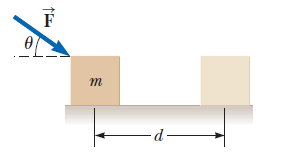# Problem: A block of mass m = 2.50 kg is pushed a distance d = 2.20 m along a frictionless, horizontal table by a constant applied force of magnitude F = 16.0 N directed at an angle θ = 25.0° below the horizontal as shown in the figure. Determine the work done on the block by(a) the applied force(b) the normal force exerted by the table(c) the gravitational force(d) the net force on the block

🤓 Based on our data, we think this question is relevant for Professor Efthimiou's class at UCF.

###### Problem Details

A block of mass m = 2.50 kg is pushed a distance d = 2.20 m along a frictionless, horizontal table by a constant applied force of magnitude F = 16.0 N directed at an angle θ = 25.0° below the horizontal as shown in the figure. Determine the work done on the block by
(a) the applied force
(b) the normal force exerted by the table
(c) the gravitational force
(d) the net force on the blockWhat scientific concept do you need to know in order to solve this problem?

Our tutors have indicated that to solve this problem you will need to apply the Net Work & Kinetic Energy concept. You can view video lessons to learn Net Work & Kinetic Energy. Or if you need more Net Work & Kinetic Energy practice, you can also practice Net Work & Kinetic Energy practice problems.

How long does this problem take to solve?

Our expert Physics tutor, Julia took 2 minutes and 39 seconds to solve this problem. You can follow their steps in the video explanation above.

What professor is this problem relevant for?

Based on our data, we think this problem is relevant for Professor Efthimiou's class at UCF.

What textbook is this problem found in?

Our data indicates that this problem or a close variation was asked in Physics for Scientists and Engineers - Serway Calc 9th Edition. You can also practice Physics for Scientists and Engineers - Serway Calc 9th Edition practice problems.